# SSC CGL Mock Tests

Since the competition for SSC exams is really tough, it is imperative for candidates to give a finishing touch to their SSC CGL preparation with the SSC CGL Online test series. The free SSC CGL mock tests provided by BYJU’s will help candidates practice and outshine in the examination.

The Staff Selection Commission conducts the SSC CGL exam. The exam is conducted for various Group B and Group C posts for various departments of the Central Government.

The SSC CGL exam is taken up by lakhs of candidates each year and the free SSC CGL mock tests will help candidates prepare for the actual exam. Each mock test consists of 25 questions from the three main sections of the SSC exams: reasoning ability, quantitative aptitude and English language.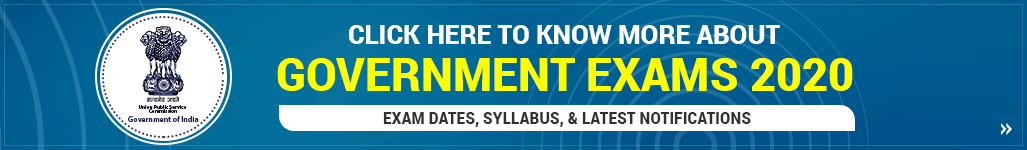### SSC CGL Exam Dates

The SSC CGL exam notification for the year 2019-20 is released on October 29th, 2019. The recruitment for the SSC CGL exam for the year 2018 was conducted in 2019. Similarly,  the recruitment for SSC CGL exam for the year 2019 will be conducted in the year 2020. The important dates for SSC CGL exam is as follows:

The table below gives a complete idea about the SSC CGL 2019 Exam:

 Name of the Recruitment Exam SSC CGL Exam 2019 -2020 Recruiting board Staff Selection Commission SSC CGL Notification Released on 22nd October 2019 SSC CGL Vacancies More than 9200(Tentative) Online Application Submission 22nd October 2019 to 25th November 2019 SSC CGL Admit Card To be released Tier I exam March 2, 2020, to March 11, 2020 Tier-II exam 22nd June 2020 to 25th June 2020 Tier III exam 22nd June 2020 to 25th June 2020 Tier IV exam To be announced

As the examination for SSC CGL 2019 Tier I is already started from March 2nd, 2020, candidates willing to appear for the next recruitment of SSC CGL exam can check the SSC CGL exam analysis for a better understanding of the scope and difficulty level of the exam.

Below Mentioned table gives the important dates for SSC CGL 2018 exam.

 SSC CGL Exam SSC CGL Tier I June 4 to June 19, 2019 SSC CGL Tier-II September 11 to September 13, 2019 SSC CGL (Descriptive) December 29, 2019

Candidates can get more details related to SSC CGL exam in the linked article.

### SSC CGL Exam Pattern

The SSC CGL exam pattern is set by the Staff Selection Commission for the different posts. The exam pattern for SSC CGL exam is given below in brief:

 SSC CGL Exam Pattern SSC CGL Tier I General Intelligence & Reasoning General Awareness Quantitative Aptitude English Language SSC CGL Tier-II Quantitative Aptitude English Language Statistics General Studies (Finance & Economics) SSC CGL Tier III English/ Hindi Descriptive Paper

Candidates must regularly give SSC CGL mock tests to analyse their preparation level. It gives candidates a chance to practice and revise the syllabus based on the actual exam pattern.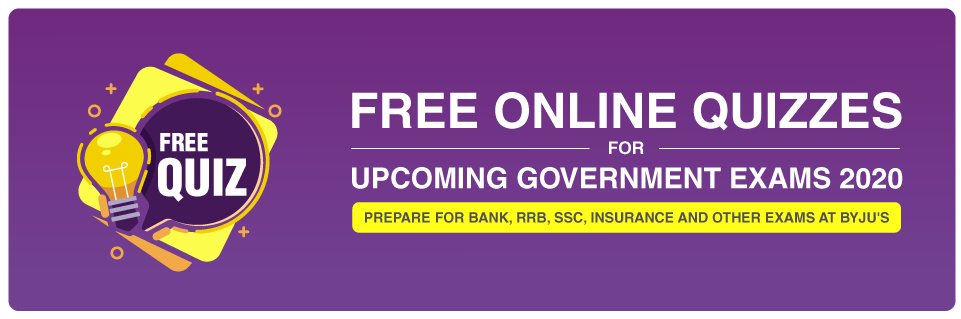## SSC CGL Free Mock Tests

The free SSC CGL mock test pattern will be the same as the one given below. Candidates can refer to the free SSC CGL mock test given below.

## SSC CGL Mock Test 1

Q. (1) In a row of girls, position of Shreya from the left side of the row is 11th and Aishwarya from the right side of the row is 17th. If Reema is sitting just in the middle of Shreya and Aishwarya and her position from Shreya is 5th, then how many girls are sitting in the row?

1. 35
2. 37
3. 26
4. 29
5. 31

Solution: Position of Reema from left = Position of Shreya from left + Position of Reema from Shreya = 11 + 5 = 16th

Position of Reema from right = Position of Aishwarya from right + Position of Shreya from Aishwarya = 17 + 5 = 22nd

Total number of girls = (Sum of the positions of Reema from both sides) – 1

= (16 + 22) – 1

= 37

Directions Q. (2-3): Read the following information carefully and answer the questions that follow:

• ‘A * B’ means ‘A is the mother of B’
• ‘A x B’ means ‘A is the father of B’
• ‘A + B’ means ‘A is the sister of B’
• ‘A – B’ means ‘A is the brother of B’
• ‘A > B’ means ‘A is the son of B’
• ‘A < B’ means ‘A is the daughter of B’

Q. (2) In the expression ‘P x Q + Y > Z’, how is Z related to P?

1. Daughter-in-law
2. Daughter
3. Wife
4. Sister
5. Brother

Solution: P x Q → P is the father of Q

Q + Y → Q is the sister of Y

Y > Z → Y is the son of Z

Hence, Z is the wife of P.

Q. (3) In the expression ‘ X + Y > M – N’, how is X related to N?

1. Son
2. Daughter
3. Nephew
4. Niece
5. Mother

Solution: X + Y → X is the sister of Y

Y > M → Y is the son of M

M – N → M is the brother of N

Therefore, X is the niece of N.

Directions Q. (4-5): In these questions, the relationship between different elements is shown in the statements. The statements are followed by two conclusions. Mark your answer as:

1. If only conclusion I is true
2. If only conclusion II is true
3. If either conclusion I or II is true
4. If neither conclusion I nor II is true
5. If both the conclusions I and II are true.

Q. (4) Statement: A > B ≥ C; D < B < E

Conclusions:

1. A > D
2. C < E

Solution: From the given statement, A > B ≥ C; D < B < E , we can conclude:

• A > B, D < B. Therefore, A > D.
• B ≥ C, B < E. Therefore, C < E.

Q. (5) Statement: P < Q = O ≥ N > M

Conclusions:

1. Q > M
2. P < N

Solution: From the given statement, P < Q = O ≥ N > M, we can conclude:

• Q = O, O > M, therefore, Q > M
• P < N

Directions Q. (6-7): Read the following statement carefully and answer the question given below.

• Point Q is 6 metres towards the North of point P.
• Point T is 10 metres towards the East of point Q.
• Point R is 7 metres towards the East of point P.
• Point S is 11 metres towards the West of point R.

Q. (6) How far should one walk from point P in order to reach point S?

1. 8 metres
2. 4 metres
3. 7 metres
4. 11 metres
5. 9 metres

Solution: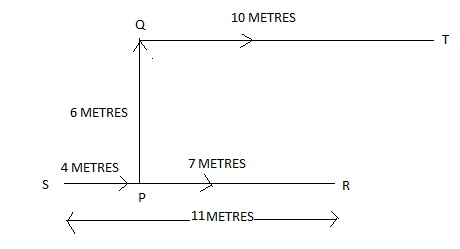Q. (7) If a person walks 6 metres South from the point T and then walks after taking a right turn, which of the following points would he reach first?

1. P
2. Q
3. R
4. S
5. None of the above

Solution: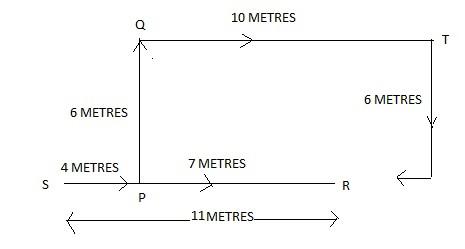Directions Q. (8): In each of the following questions, a question is followed by two statements. You have to select a statement that answers the question. Mark your answer as:

1. If the data in statement I alone is sufficient to answer the question while the data in statement II alone is not sufficient to answer the question.
2. If the data in statement II alone are sufficient to answer the question while the data in statement I alone is not sufficient to answer the question.
3. If the data either in statement I alone or in statement II alone is sufficient to answer the question.
4. If the data in both statements I and II together are not sufficient to answer the question.
5. If the data in both statements I and II together are necessary to answer the question.

Q. (8) Six girls A, B, C, D, E and F are sitting around a circular table with an equal distance between them. If all the girls are facing the centre, then who is sitting opposite to F?

Statements:

1. F is sitting between A and D while E is sitting adjacent to B and D.
2. A is adjacent to both F and C while B is sitting adjacent to C.

Answer: c (If the data either in statement I alone or in statement II alone is sufficient to answer the question)

Solution: As per the question, we get the following two arrangements: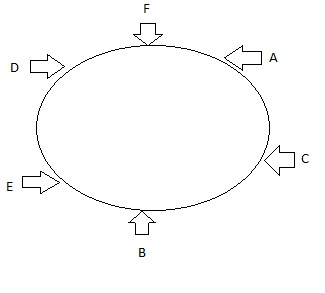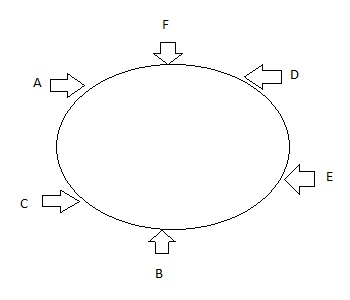Therefore, either from statement I or statement II alone, we can conclude that B is sitting opposite to F. Thus, the data either in statement I alone or in statement II alone are sufficient to answer the question.

Directions Q. (9-11): What will come in place of (?) in the given series?

Q. (9) 22, 23, 27, 36, 52, 77, (?)

1. 92
2. 96
3. 113
4. 117
5. 120

Solution: The pattern of the given series is as follows:

• 22 + 12 = 23
• 23 + 22 = 27
• 27 + 32 = 36
• 36 + 42 = 52
• 52 + 52 = 77
• 77 + 62 = 113

Q. (10) 5, 30, 185, 1300, (?), 93650

1. 10200
2. 10405
3. 5450
4. 7800
5. 7650

Solution: The pattern of the given series is:

• (5 x 5) + 5 = 30
• (30 x 6) + 5 = 185
• (185 x 7) + 5 = 1300
• (1300 x 8) + 5 = 10405
• (10405 x 9) + 5 = 93650

Q. (11) 330, 547, 673, 738, 766, (?)

1. 775
2. 873
3. 947
4. 830
5. 780

Solution: The pattern of the given series is:

• 330 + 63 + 1 = 547
• 547 + 53 + 1 = 673
• 673 + 43 + 1 = 738
• 738 + 33 + 1 = 766
• 766 + 23 + 1 = 775

Directions Q. (12-15): The table given below shows the ratio between literate and illiterate persons and the population of seven villages of a district. Study the table and select the most appropriate answers.

 Villages Ratio (literate: illiterate) Population A 9: 2 2354 B 4: 1 2540 C 7: 2 2709 D 13: 4 5100 E 5 : 3 3248 F 5: 2 4921 G 13: 6 3990

Q. (12) If 42% of the illiterates of village D are males, then what is the number of illiterate women in this village?

1. 600
2. 696
3. 750
4. 830
5. 800

Solution: Number of illiterates in village D = (4 / 17) x 5100 = 1200

Number of illiterate females in village D = (100 – 42) % of 1200

= 58% of 1200 = (58 / 100) x 1200 = 696

Q. (13) If the ratio between males and females in the village G is 11: 8 and 60% females in village G are illiterates, then how many males of this village are illiterate?

1. 200
2. 252
3. 320
4. 380
5. 170

Solution: Number of literates in village G = (8 / 19) x 3990 = 1680

Number of illiterate women = 60% of 1680 = (60 / 100) x 1680 = 1008

Number of illiterates in village G = (6 / 19) x 3990 = 1260

Therefore, number of illiterate males in village G = 1260 – 1008 = 252

Q. (14) What is the difference between the number of literates in Village C and the number of illiterates in Village A?

1. 1500
2. 1679
3. 1342
4. 230
5. 1670

Solution:

Number of literates in Village C = 7/9 x 2709 = 2107

Number of illiterates in Village A = 2/11 x 2354 = 428

Difference between the number of literates in Village C and the number of illiterates in Village A = 2107 – 428 = 1679

Q. (15) By what percentage is the number of literates in village F is more than the number of literates in village A?

1. 80%
2. 60%
3. 82.50%
4. 88%
5. 75%

Solution: Number of literates in village F = (5 / 7) x 4921 = 3515

Now, the number of literates in village A = (9 / 11) x 2354 = 1926

Therefore, required percentage = [(Number of literates in village F – Number of literates in village A) / Number of literates in village A] x 100

= [(3515 – 1926) / 1926] x 100

= 82.50

Directions Q. (16 -17): In each question, two equations numbered I and II are given. You have to solve both the equations and mark answer as

1. If p < q
2. If p > q
3. If p ≥ q
4. If p ≤ q
5. If p = q

Q. (16) I. p = (729)

II. q2 = 7225, q > 0

Solution: Equation I ⇒ p = (93)

⇐ p = (9)3 x ⅔ = (9)2 = 81

Equation II ⇒ q2 = (± 85)2

⇐ q = ± 85

But since q > 0, therefore, q = 85

Hence, p < q

Q. (17) I. p2 – 22p + 121 = 0

II. q2 = (1331)

Solution: Equation I ⇒ p2 – 11p – 11p + 121 = 0

⇐ p(p – 11) – 11(p – 11) = 0

⇐ (p – 11) (p – 11) = 0

⇐ p = 11

Equation II ⇒ q2 = (113)

⇐ q2 = (11)2

⇐ q = 11

Therefore, p = q

Directions Q. (18-20): Find the antonyms of each of the words given in bold in the following questions.

Q. (18) Churn

1. Agitate
2. Seethe
3. Heave
4. Embed
5. Baffle

Q. (19) Propel

1. Mobilize
2. Sling
3. Flip
4. Shy
5. Repress

1. Wane
2. Dim
3. Slack off
4. Strengthen
5. Dwindle

Directions Q. (21-23): Read each sentence to find out whether there is any grammatical error in it. The error, if any, will be in one part of the sentence marked as (1), (2), (3), (4) and (5). If the sentence is correct, mark option (5) as your answer.

Q. (21) Ridha picked them up one by one (1) / and admired craftsmanship (2) / and then saw that there (3) / were a note in the box as well. (4) / No error (5)

1. (1)
2. (2)
3. (3)
4. (4)
5. (5)

Q. (22) The Chief Minister of Assam sits (1) / third to the right of the (2) / Chief Minister of Delhi and (3) / neither of them sits at extreme ends. (4) / No error (5)

1. (1)
2. (2)
3. (3)
4. (4)
5. (5)

Q. (23) The poor landless (1) / and marginal farmers (2) / have no other choice (3) / than migrating. (4) / No error (5)

1. (1)
2. (2)
3. (3)
4. (4)
5. (5)

Directions Q. (24-25): Replace the bold word with an appropriate and synonymous word in each of the following questions.

Q. (24) On 21st September every year, World Peace Day is observed. This day was declared by the United Nations General Assembly to strengthen the ideals of peace.

1. Invigorate
2. Curtail
3. Vigilante
4. Improve
5. Liberate

Q. (25) Snowy fortunately became the heir to a huge palace and acquired the power to rule the kingdom.

1. Devisee
2. Hermit
3. Ere
4. Misanthrope
5. Anchorite

Candidates must check this page regularly for new SSC CGL free Mock Tests.## Frequently Asked Question -SSC CGL Online Mock Tests

### Q.1. Does BYJU’S provide solutions to SSC CGL Free Mock Tests?

Ans. Yes, each online mock test of SSC CGL gives the answer and detailed solutions to the questions.

### Q.2. How to prepare for SSC CGL exam in 3 months?

Ans. To prepare effectively for the SSC CGL Exam one must have a lot of reference books handy. These books help them focus better on the subject matter and ultimately score well in the exam. Some important subject wise SSC CGL Books along with useful preparation tips are given in the SSC CGL Preparation page.

### Q.3. Can a candidate retake the SSC CGL practice papers to improve his score?

Ans. As the Free SSC CGL online mock tests are available in PDF format, candidates can download the pdf and solve the SSC CGL sample papers offline to practice and improve.

### Q.4. Why should I choose BYJU’S SSC CGL online mock tests series?

Ans. BYJU’S SSC CGL Mock Tests are designed by the experts with an aim to provide the best preparation for SSC Combined Graduate Level 2020 exam. The full length and section-wise SSC CGL online mock tests simulate the real exam scenario. The detailed solution of all the questions after each practice paper gives performance reports and thus helps candidates to work on the weak sections to score better marks in the final exams.

### Q 5. How can a candidate choose the right SSC CGL Sample Papers?

Ans. The digital resource can help candidates find out various SSC CGL free online mock tests or sample papers, but they must clarify which mock papers will be good enough to help them improve their quality of performance in the SSC CGL 2020 exam. Here the parameters to choose right SSC CGL sample papers or online test series:

• Firstly, ensure the SSC CGL mock tests are aligned with the latest exam pattern and syllabus.
• The level of questions included in the SSC CGL practice papers must be moderate.
• The free mock tests for SSC CGL must have standardised and unique questions.
• The SSC CGL practice papers must be easily accessible without any technical issues.
• The analysis report of SSC CGL Online Test Series must give clarity on weaknesses and strengths with regards to the SSC CGL Syllabus.

### Q.6. Can a candidate attempt SSC CGL Online Test series offline also?

Ans. Yes, candidates can download the SSC CGL Mock Tests PDF and can attempt it offline as many times as they want.